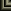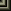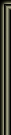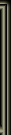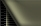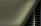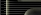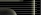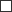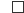Oops I broke Nektulos..
 Home Forums Register Members List Search Today's Posts Mark Forums ReadSupport::Windows Servers Support forum for Windows EQEMu users.#1
 jsr Hill Giant Join Date: Aug 2008 Location: melbourne Posts: 171Oops I broke Nektulos..

I was editing zone points in Nektulos, I zoned out, zoned in, changed a co-ordinate, #reloadzps.... and suddently no zone points are working in the zone. It's as if there are no zone points.

I reset server and client, same problem.
I deleted all of the nektulos zone points from the DB and reloaded them from PEQ, same problem.
I tried a fresh client install, same problem.

Anyone come across this before?#2
 Huppy Dragon Join Date: Oct 2010 Location: Canada Posts: 957I'm sure sure your aware there are 2 versions of nektulos in the zone table, (old and new) - ID 439 (version 1) ID 246 (version 0) The following is something you can either use to restore, or use for reference:

(This is assuming these zone_points exist in your DB)

These are all the default zone_points going OUT of Nektulos

Code:
```UPDATE `zone_points` SET `zone` = 'nektulos', `version` = 1, `number` = 20, `y` = 0, `x` = 0, `z` = 0, `heading` = 0, `target_y` = -386, `target_x` = -133, `target_z` = -64, `target_heading` = 145, `zoneinst` = 0, `target_zone_id` = 365, `target_instance` = 0, `buffer` = 0, `client_version_mask` = 4294967292 WHERE `id` = 1477;
UPDATE `zone_points` SET `zone` = 'nektulos', `version` = 1, `number` = 10, `y` = 2325, `x` = 740, `z` = 56, `heading` = 0, `target_y` = 118, `target_x` = -18, `target_z` = -62, `target_heading` = 506, `zoneinst` = 0, `target_zone_id` = 27, `target_instance` = 0, `buffer` = 0, `client_version_mask` = 4294967292 WHERE `id` = 1476;
UPDATE `zone_points` SET `zone` = 'nektulos', `version` = 1, `number` = 30, `y` = 0, `x` = 0, `z` = 0, `heading` = 0, `target_y` = 1355, `target_x` = 798, `target_z` = -18, `target_heading` = 87, `zoneinst` = 0, `target_zone_id` = 22, `target_instance` = 0, `buffer` = 0, `client_version_mask` = 31 WHERE `id` = 1478;
UPDATE `zone_points` SET `zone` = 'nektulos', `version` = 1, `number` = 77, `y` = 0, `x` = 0, `z` = 0, `heading` = 0, `target_y` = -841, `target_x` = 132, `target_z` = -157, `target_heading` = 257, `zoneinst` = 0, `target_zone_id` = 202, `target_instance` = 0, `buffer` = 0, `client_version_mask` = 4294967292 WHERE `id` = 1480;
UPDATE `zone_points` SET `zone` = 'nektulos', `version` = 1, `number` = 30, `y` = 0, `x` = 0, `z` = 0, `heading` = 0, `target_y` = 1292, `target_x` = -2480, `target_z` = 78, `target_heading` = 101.5, `zoneinst` = 0, `target_zone_id` = 408, `target_instance` = 0, `buffer` = 0, `client_version_mask` = 4294967232 WHERE `id` = 2339;
UPDATE `zone_points` SET `zone` = 'nektulos', `version` = 1, `number` = 50, `y` = 0, `x` = 0, `z` = 0, `heading` = 0, `target_y` = 53, `target_x` = 154, `target_z` = 28, `target_heading` = 251, `zoneinst` = 0, `target_zone_id` = 40, `target_instance` = 0, `buffer` = 0, `client_version_mask` = 4294967292 WHERE `id` = 1479;
UPDATE `zone_points` SET `zone` = 'nektulos', `version` = 0, `number` = 1, `y` = 0, `x` = 0, `z` = 0, `heading` = 0, `target_y` = 53, `target_x` = 154, `target_z` = 28, `target_heading` = 130, `zoneinst` = 0, `target_zone_id` = 40, `target_instance` = 0, `buffer` = 0, `client_version_mask` = 4294967295 WHERE `id` = 1444;
UPDATE `zone_points` SET `zone` = 'nektulos', `version` = 0, `number` = 2, `y` = -2702, `x` = -654, `z` = -18, `heading` = 0, `target_y` = 1549, `target_x` = 627, `target_z` = -20, `target_heading` = 110, `zoneinst` = 0, `target_zone_id` = 22, `target_instance` = 0, `buffer` = 0, `client_version_mask` = 4294967295 WHERE `id` = 1445;
UPDATE `zone_points` SET `zone` = 'nektulos', `version` = 0, `number` = 3, `y` = 0, `x` = 0, `z` = 0, `heading` = 0, `target_y` = 81.43, `target_x` = -10.38, `target_z` = -54.94, `target_heading` = 0, `zoneinst` = 0, `target_zone_id` = 27, `target_instance` = 0, `buffer` = 0, `client_version_mask` = 4294967295 WHERE `id` = 1446;
UPDATE `zone_points` SET `zone` = 'nektulos', `version` = 0, `number` = 77, `y` = 0, `x` = 0, `z` = 0, `heading` = 0, `target_y` = -841, `target_x` = 132, `target_z` = -157, `target_heading` = 1, `zoneinst` = 0, `target_zone_id` = 202, `target_instance` = 0, `buffer` = 0, `client_version_mask` = 4294967295 WHERE `id` = 1447;```
These are all the default zone_points going IN to Nektulos:

Code:
```UPDATE `zone_points` SET `zone` = 'ecommons', `version` = 0, `number` = 4, `y` = 1553, `x` = 641, `z` = -18, `heading` = 0, `target_y` = -2679.36, `target_x` = -514.8, `target_z` = -19.34, `target_heading` = 50, `zoneinst` = 0, `target_zone_id` = 25, `target_instance` = 0, `buffer` = 0, `client_version_mask` = 3 WHERE `id` = 53;
UPDATE `zone_points` SET `zone` = 'neriaka', `version` = 0, `number` = 3, `y` = 29, `x` = 155, `z` = 30, `heading` = 0, `target_y` = 2299.79, `target_x` = -1108.24, `target_z` = 2.19, `target_heading` = 64, `zoneinst` = 0, `target_zone_id` = 25, `target_instance` = 0, `buffer` = 0, `client_version_mask` = 3 WHERE `id` = 415;
UPDATE `zone_points` SET `zone` = 'poknowledge', `version` = 0, `number` = 17, `y` = 0, `x` = 0, `z` = 0, `heading` = 0, `target_y` = 705, `target_x` = -349, `target_z` = -4, `target_heading` = 20, `zoneinst` = 0, `target_zone_id` = 25, `target_instance` = 0, `buffer` = 0, `client_version_mask` = 3 WHERE `id` = 468;
UPDATE `zone_points` SET `zone` = 'lavastorm', `version` = 0, `number` = 0, `y` = 75, `x` = -10, `z` = -53, `heading` = 0, `target_y` = 3107, `target_x` = 289, `target_z` = -17, `target_heading` = 88, `zoneinst` = 0, `target_zone_id` = 25, `target_instance` = 0, `buffer` = 0, `client_version_mask` = 3 WHERE `id` = 658;
UPDATE `zone_points` SET `zone` = 'corathus', `version` = 0, `number` = 1, `y` = 999999, `x` = 999999, `z` = 999999, `heading` = 999, `target_y` = -697.9, `target_x` = 1271.2, `target_z` = -2.2, `target_heading` = 169.5, `zoneinst` = 0, `target_zone_id` = 25, `target_instance` = 0, `buffer` = 0, `client_version_mask` = 4294967295 WHERE `id` = 1458;
UPDATE `zone_points` SET `zone` = 'corathus', `version` = 0, `number` = 30, `y` = 0, `x` = 0, `z` = 0, `heading` = 0, `target_y` = -1026, `target_x` = 1187, `target_z` = -11, `target_heading` = 442, `zoneinst` = 0, `target_zone_id` = 25, `target_instance` = 0, `buffer` = 0, `client_version_mask` = 4294967295 WHERE `id` = 1501;
UPDATE `zone_points` SET `zone` = 'commonlands', `version` = 0, `number` = 20, `y` = 0, `x` = 0, `z` = 0, `heading` = 0, `target_y` = -2192, `target_x` = -419, `target_z` = 2, `target_heading` = 235, `zoneinst` = 0, `target_zone_id` = 25, `target_instance` = 1, `buffer` = 0, `client_version_mask` = 4294967295 WHERE `id` = 1575;
UPDATE `zone_points` SET `zone` = 'ecommons', `version` = 0, `number` = 4, `y` = 1553, `x` = 641, `z` = -18, `heading` = 0, `target_y` = -2225.7, `target_x` = -466.5, `target_z` = 3.6, `target_heading` = 246.8, `zoneinst` = 0, `target_zone_id` = 25, `target_instance` = 1, `buffer` = 0, `client_version_mask` = 4294967292 WHERE `id` = 1585;
UPDATE `zone_points` SET `zone` = 'neriaka', `version` = 0, `number` = 3, `y` = 29, `x` = 155, `z` = 30, `heading` = 0, `target_y` = 1824.4, `target_x` = -977.1, `target_z` = 24.7, `target_heading` = 71.5, `zoneinst` = 0, `target_zone_id` = 25, `target_instance` = 1, `buffer` = 0, `client_version_mask` = 4294967292 WHERE `id` = 1586;
UPDATE `zone_points` SET `zone` = 'poknowledge', `version` = 0, `number` = 17, `y` = 0, `x` = 0, `z` = 0, `heading` = 0, `target_y` = -814.6, `target_x` = -833.7, `target_z` = 9.9, `target_heading` = 218.8, `zoneinst` = 0, `target_zone_id` = 25, `target_instance` = 1, `buffer` = 0, `client_version_mask` = 4294967292 WHERE `id` = 1587;
UPDATE `zone_points` SET `zone` = 'lavastorm', `version` = 0, `number` = 0, `y` = 75, `x` = -10, `z` = -53, `heading` = 0, `target_y` = 2275.93, `target_x` = 744.19, `target_z` = 38, `target_heading` = 128, `zoneinst` = 0, `target_zone_id` = 25, `target_instance` = 1, `buffer` = 0, `client_version_mask` = 4294967292 WHERE `id` = 1588;
UPDATE `zone_points` SET `zone` = 'corathus', `version` = 0, `number` = 1, `y` = 999999, `x` = 999999, `z` = 999999, `heading` = 999, `target_y` = -1053.9, `target_x` = 1193.9, `target_z` = -4.2, `target_heading` = 221.3, `zoneinst` = 0, `target_zone_id` = 25, `target_instance` = 1, `buffer` = 0, `client_version_mask` = 4294967292 WHERE `id` = 1589;```
__________________
Don't hate the dev...hate the game ....#3
 jsr Hill Giant Join Date: Aug 2008 Location: melbourne Posts: 171Thanks Huppy,

Resetting the zone points isn't working for some reason. I've reverted to the original zone, which is working, but the underlying issue is a mystery for now.#4
 Huppy Dragon Join Date: Oct 2010 Location: Canada Posts: 957Quote:
 Originally Posted by jsr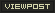I've reverted to the original zone, which is working, but the underlying issue is a mystery for now.
One of my little home projects I am working on, I am using the older classic version of nektulos. It was a little tedious getting all the zone points going in the right direction, but I never encountered the issues you're having. I would be curious though, to find out what caused it, just for future reference, in case someone else runs into the same thing and the info could be passed along.
__________________
Don't hate the dev...hate the game ....Thread ToolsShow Printable VersionEmail this Page Display ModesLinear ModeSwitch to Hybrid ModeSwitch to Threaded Mode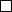Posting Rules You may not post new threads You may not post replies You may not post attachments You may not edit your posts BB code is On Smilies are On [IMG] code is On HTML code is Off Forum Rules
 Forum Jump User Control Panel Private Messages Subscriptions Who's Online Search Forums Forums Home General     General::General Discussion     General::Server Discussion     General::News         General::News::Polls Support     Support::General Support         Idiot Posters     Spell Support     Support::Windows Servers     Support::Linux Servers     Support::Packetcollector     Support::MiniLogin Quests     Quests::Q&A     Quests::Custom     Quests::Completed     Quests::Submissions     Quests::Plugins & Mods     Quests::need work     Quests::LUA Development     Development::Development     Development::Bots     Development: Custom Code     Development::Server Code Submissions     Development::Feature Requests     Development::Bugs     Development::Bug Reports     Development::Database/World Building     Development::Tools     Development::GeorgeS's Tools     EQEmu Operations Center     KayotRO     Old Eq OpenZone     OpenZone:: Q&A     Simple Client     OpenZone::Tutorials     OpenZone::Bug Reporting Misc     Misc::Off Topic     Misc::Screenshots Archives     Archive::Support         Archive::General Support         Archive::Windows Servers         Archive::Linux Servers         Archive::Tutorials/Howto's             Tutorials--Outdated Use the Wiki                 Tutorial ALL NEW PEOPLE READ THIS                 Tutorials::Discussion                 Tutorials::Requests                 Tutorials::Submissions                 Tutorials::Needs work         Archive::Packetcollector     Archive::Development         Archive::Development         Archive::Bugs         Archive::Tools         Archive::Quests         Archive::Database/World Building         EQ2 Development     Archive::Misc         Archive::Off Topic         Archive::Screenshots     OpenEQ         OpenEQ::General Discussion         OpenEQ::Support         OpenEQ::Development         OpenEQ::Bug reporting         OpenEQ::Plugins

All times are GMT -4. The time now is 09:39 PM.

 -- Default Style ---- EQEmulator Contact Us - EQEmulator - Archive - Top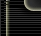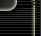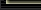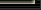Everquest is a registered trademark of Daybreak Game Company LLC. EQEmulator is not associated or affiliated in any way with Daybreak Game Company LLC. Except where otherwise noted, this site is licensed under a Creative Commons License. Powered by vBulletin®, Copyright ©2000 - 2020, Jelsoft Enterprises Ltd. Template by Bluepearl Design and vBulletin Templates - Ver3.3